AP Physics B : Forces

Example Questions

2 Next →

Example Question #11 : Forces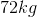astronaut on Earth uses a space elevator to get to a space station. From the ground, the elevator accelerates upwards at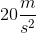. During this acceleration, what is the normal force acting on the astronaut?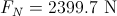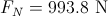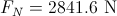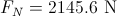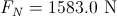Explanation:

This question essentially asks how much the astronaut will weigh during acceleration. The two forces acting on the astronaut are the downward gravitational force and the upward normal force. The net force acting on this astronaut is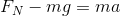.

Notice that the net force is set to equal to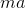to show that the astronaut is accelerating due to the elevator.

Isolate the normal force.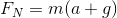Use the given values to solve.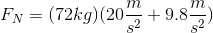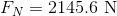Example Question #11 : Ap Physics 1

A potted plant is hanging from a rope attached to a hook. The plant exerts 25N of force. Assume the rope is weightless.

If the packaging says the rope is able to withstand 800N of force, what is the maximum amount of mass that can be hanging from the rope?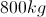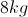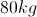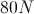Explanation:

If the rope can only withstand 800N of force, we can solve for the mass of the object which would result in this maximum force when hanging from the rope.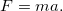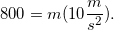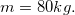Example Question #3 : Newton's Second Law

A skydiver with a mass of 100kg jumps out of an airplane and reaches a terminal velocity of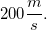.

Which of the following factors will not decrease the total force on the man as he jumps from the plane?

The man jumps as the plane is descending sharply

The man immediately opens his parachute

The man orients his stomach toward the ground, rather than his feet

Increasing the man's mass

Increasing the man's mass

Explanation:

The equation for force isThis shows that by increasing the man's mass, his force will increase after jumping from the plane.

All other options will result in a decreased acceleration when falling. Falling with his stomach downward increases the surface area of the diver, which increases his air resistance. A parachute will also increase the air resistance and surface area. If the plane is descending when he jumps, his time to reach terminal velocity will be reduced, as he will already have an initial velocity in the downward direction.

Example Question #404 : Mcat Physical Sciences

A skydiver with a mass of 100kg jumps out of an airplane and reaches a terminal velocity of.

What is the total force acting on the skydiver at this point in his jump?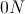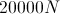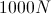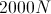Explanation:

The man has reached a terminal velocity, which means that he has an acceleration of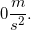Because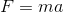, the total force on the skydiver is 0N. At this point, the force due to gravity in the downward direction is equal to the force of air resistance in the upward direction.

Example Question #1 : Understanding Newton's Laws

A man pushes a crate across a frictionless floor at a constant velocity. Which of the following statements is true?

The net force on the man is greater than zero

The force on the crate is less than the force on the man

The force the man exerts on the crate is equal and in the same direction as the force the crate exerts on the man

The force the man exerts on the crate is equal and opposite to the force the crate exerts on the man

The force on the crate is greater than the force on the man

The force the man exerts on the crate is equal and opposite to the force the crate exerts on the man

Explanation:

According to Newton's third law, the force on the crate must have the same magnitude as the force on the man. The forces must also be in opposite directions. We can conclude that the net force on the man is zero, since he is moving with the crate at a constant velocity.

Example Question #2 : Newton's Second Law

A 2000kg car with a velocity of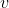collides head on with a 6000kg truck with a velocity of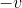. Which vehicle experiences the greater force? Which experiences the greater acceleration?

The truck experiences the greater force and the car experiences the greater acceleration

The truck experiences the greater force and the greater acceleration

Both the car and the truck experience equal force and acceleration

The car experiences the greater force and the greater acceleration

The car and the truck experience equal force and the car experiences greater acceleration

The car and the truck experience equal force and the car experiences greater acceleration

Explanation:

The car and the truck experience equal and opposite forces, but since the car has a smaller mass it will experience greater acceleration than the truck according to the equation F = ma.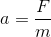A greater mass will decrease the acceleration.

Example Question #3 : Universal Gravitation

The moon's distance from the center of the Earth was decreased by a multiple of three. How would this affect the gravitational force of the Earth on the moon?

It would increase by a factor of three

It would decrease by a factor of nine

It would increase by a factor of nine

The gravitational force would not be affected, because the moon's mass is the same

It would increase by a factor of nine

Explanation:

The law of gravitation is written as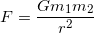, with G being equal to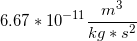.

Since the radius of the two masses acting on each other is squared, and is found in the denominator, a decrease in the radius by a multiple of three will cause a nine-fold increase in the gravitational force.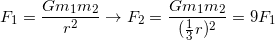Example Question #1 : Understanding Gravity And Gravitational Forces

What is the acceleration due to gravity on a planet on which an object with a mass of 20.0kg has a weight of 270N?

27m/s2

0.074m/s2

13.5m/s2

10m/s 2

13.5m/s2

Explanation:

Solve the following equation for acceleration, using the values given in the question.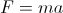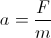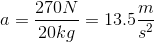Example Question #1 : Motion In One DimensionA ball is thrown horizontally off a cliff of height of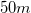with an initial velocity of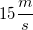. How far from the cliff will the ball land?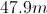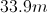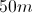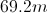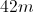Explanation:

First we will find the time required for the ball to reach the ground. Since the ball is thrown horizontally, it has no initial vertical component. We use the following equation to solve for the total flight time: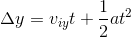We are given the change in height, initial velocity, and acceleration. Using these values, we can solve for the time. Note that the change in height will be negative, since the ball is traveling downward.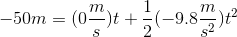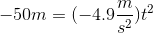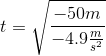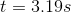Finally, we use the horizontal velocity to find the distance traveled in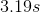. Remember that the horizontal velocity remains constant during projectile motion.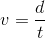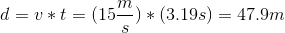Example Question #1 : Understanding Gravity And Gravitational Forces

A certain planet has three times the radius of Earth and nine times the mass. How does the acceleration of gravity at the surface of this planet (ag) compare to the acceleration at the surface of Earth (g)?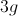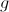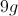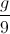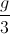Explanation:

The acceleration of gravity is given by the equation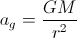, where G is constant.

For Earth,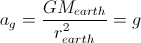.

For the new planet,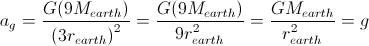.

So, the acceleration is the same in both cases.

2 Next →